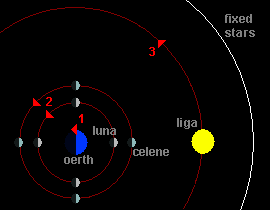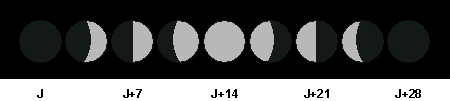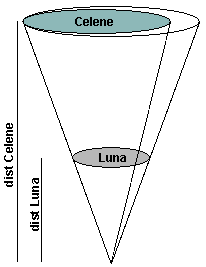It is well known that Oerth is the center of the Primary Plane. There are 3 massive bodies around it: The sun Liga, and the two moons Luna and its small sister Celene.

*Shadowwise is the way the shadows rotate. It is sometimes called clockwise – word from an unknown origin.The Flanaess Tides Mathematical Model: Moon Locations

Liga rises in the East and sets in the West. This is due to the rotation of Oerth on itself. So 1 is counter-shadowwise.

Both moons have the following phases:The Flanaess Tides Mathematical Model: Moon Phases

The sun is almost motionless compared to the moon’s cycles so 2 is counter-shadowwise too.

The visible Fixed Stars change along the year. Constellations set and rise earlier every day. Each month, before sunrise, a new zodiac constellation can be seen on the East of the previous ones (the left in the north hemisphere). So that 3 is counter-shadowwise too.

## Periods

Seen from Oerth, all look simple. The planet rotates around itself in exactly one day, Luna has a cycle of 28 days, Celene has a cycle of 91 days, and Liga’s one is 364 days. However, the visible cycle – aka the synodic period – of the moons is relative to Liga’s position, and it changes around the year.

If we consider Celene for example, two full moons are separated by 91 days.But during that time, Liga made a quarter of its cycle, so that in fact Celene’s real move in 91 days is 1.25 turns. Finally, Celene turns around earth 5 times a year while we see only 4 cycles:

In the very same way, Luna rotates Oerth 14 times a year, that is 1+364/28.

The Third Kepler Law defines a relation between the distances and the periods: Pluna² / Pcelene² = dluna³ / dcelene³
The Period of Luna is Pluna = 1/14
The Period of Celene is Pcelene = 1/5

That is dcelene = (Pcelene/Pluna)2/3 * dluna = (14/5)2/3 * dluna = 1.987 dluna

So that Celene is about twice as far as Luna.

It is well known that Celene looks a little smaller than Luna in the sky. However, Celene visible diameter is not very small since it can cause sun eclipses too. Let’s imagine a moment that Celene actually looks 80% the size of Luna.Celene is twice as far as Luna; so that Thales teaches us that about radiuses (real ones, not visible ones): Rcelene = 80% of 2 * Rluna

In other words, Celene is actually 1.6 times bigger in diameter than Luna (even if it looks smaller seen from Oerth)

A sphere volume depends on the cube of its radius (V=4Πr³/3) so that Celene volume is 1.6³ bigger. That is Celene is 4 times bigger than Luna.

Let’s try to guess the mass of Celene. We know its volume is about 4 times Luna’s one. But we don’t know anything about its density.

If we accept the (big) assumption that the density of the two moons is roughly the same, the mass of Celene is 4 times the one of Luna.

Here are other possibilities:

 Celene visible radius (/Luna)R Celene density (/Luna)D Celene mass (/Luna)(14/5)²*R³*D 90% 1.0 5.71536 80% 1.0 4.01408 70% 1.0 2.68912 60% 1.0 1.69344 50% 1.0 0.98 80% 0.5 2.00704 80% 0.75 3.01056 80% 1.0 4.01408 80% 1.5 6.02112 80% 2.0 8.02816

Now, if we use the Law of Universal gravitation Fg=G*m1*m2/r²

For Luna, we have
Fluna = G*mOerth*mluna/dluna²

And for Celene
Fcelene = G*mOerth*mcelene/dcelene² = G*mOerth*(15.6*mluna)/(2*dluna)² = 1.6³/2² * Fluna = 1.02 * Fluna

In other words, the gravitational effect of Celene is about the same as Luna’s one.

## Tides basics

Tides exist because the gravitational force depends on the distances, and Oerth is not really a point: The closest a point is to amass, the strongest the attraction.In the scheme above, the gravitational field of Luna is stronger at point 3 than at point 2, and stronger at point 2 than at point 1.

The tidal force depends on the gradient of the gravitational field. Suppose another piece of matter is k centimetres farther in the same direction. Then it undergoes a smaller acceleration: Gm/(d+k)². The difference between these two accelerations can be ascribed to a tidal force pulling the two pieces apart. The estimation of this difference is a classic example of the linear approximation f(x+k) – f(x) ~ k f'(x). It yields -2 G m k / d³.

In other words, tides don’t react the square of the distance but to its cube. Another important consequence is that a body such as Luna causes 2 high tides: One on each side of Oerth. That’s why there are two high tides and two low tides each day.

## Tidal strengths

Luna tidal strength is TSLuna = -2 G mOerth mLuna / dLuna³

Celene tidal strength is TSCelene = -2 G mOerth mCelene / dCelene³
But we know mCelene = (14/5)² * (Visible radiusCelene / Visible radiusLuna)³ * (Density Celene / Density Luna) * mLuna
And dCelene = (14/5)2/3dLuna
that means that dCelene³ = (14/5)²dLuna
After simplification:
TSCelene = (Visible radiusCelene / Visible radiusLuna)³ * (Density Celene / Density Luna) * TSLuna

On Earth, the sun’s tidal force is 45% the one of the Moon. Luna is usually considered on Oerth as the equivalent of the Moon on Earth, so I’ll keep these numbers for tide computation.

If we keep the above hypothesis that Celene visible radius is 80% Luna’s one and that the densities are the same ones, we have the following tidal strengths ratio TSCelene = 0.512 * TSLuna

Here are the possibilities.

 Celene visible radius (/Luna)R Celene density (/Luna)D Sun strengthCSun = 1 Luna strengthCLuna = 2.22 Celene strengthCCelene = R³ * D * CLuna 100% 1.0 1.00 2.22 2.22 90% 1.0 1.00 2.22 1.62 80% 1.0 1.00 2.22 1.14 70% 1.0 1.00 2.22 0.76 60% 1.0 1.00 2.22 0.48 50% 1.0 1.00 2.22 0.28

## First model

In a first approximation, let’s imagine that Luna, Celene and Liga are all rotating in the same plane.

So we have the following tidal forces:

• sun (coef 1.0) (2 cycles in 1 day seen from Oerth, Oerth reference)
• Luna (coef 2.22) (2 cycles in 28 days seen from Oerth, geocentric reference)
• Celene (coef 1.14) (2 cycles in 91 days seen from Oerth, geocentric reference)The biggest tide is when all three pulling forces are aligned. That event occurs only once a year, that is Richfeast the 4th, at noon. That day is called Sealords’ feast in Greyhawk. (amazing, hu?)The height of the water on a given hour h can be approximated by that very basic equation
t = h4Π/24
height = 1*cos(t) + 2.22*cos(t-t/28) + 1.14*cos(t-t/91)
Where h can simply be the number of hours since noon Richfeast, the 4th.The perfect timing of these three masses revolution means that the water height is the same every year. That model works pretty well if you are near the equator.

## Latitude Influence

In order to get a better model, we have to take into account the latitude of the observer.

On the equator, the moons do reach the zenith. Their altitude varies between +90° (zenith) and -90° (nadir). However, that amplitude becomes smaller and smaller when you go toward a pole. When you reach a pole, the moons circle the horizon on a 0 altitude.

Let’s define t as the number of days this Sealords’ feast, noon. We have these figures:
AltSun = (Π/2-latitude) * cos(2*Π*t)
AltLuna = (latitude – Π/2) * cos(2*Π*t*27/28)
AltCelene = (latitude – Π/2) * cos(2*Π*t*90/91)

The total impact of each body is maximal when the angles reach the zenith or nadir and are null when the body is on the horizon:
Effectx = 1/2 – cos(Altitudex*2)/2

Finally, using the strenght computed above, we can approximate the water height:
H(t) = 1.0 * EffectSun + 2.22 * EffectLuna + 1.14 * EffectCelene

## Sun height variation around the year (seasons)

Up to now, we consider that the sun’s maximal altitude in the sky was Π/2-latitude. However, there is a significant variation. Liga doesn’t rotate in the sky like the moons around the equator. Its height follows the yearly variation of the fixed stars.

Let’s call tropic the semi-yearly variation of the fixed stars angle. The sun height additional angle variation is tropic*cos(2*Π*t/364)).

As noted in the 1983 Glossography, page 20, the 60 degrees north latitude line is Oerth’s “arctic circle,” north of which you get the phenomenon of the midnight sun. (The same thing happens, of course, south of the 60 degrees south latitude line.) As a result of this increased tilt, Oerth should actually have more severe seasonal changes in climate than Earth does. Gods and magic can alter this, of course, but somewhere on Oerth it might get very hot in the summer and very cold in the winter.

## Finally

If t is the number of days since noon Sealords’ Feast, latitude the latitude of the observer on Oerth:

 AltSun(t) = (Π/2-latitude) * cos(2*Π*t) + Π/6*cos(2*Π*t/364))AltLuna(t) = (latitude – Π/2) * cos(2*Π*t*27/28)AltCelene(t) = (latitude – Π/2) * cos(2*Π*t*90/91)Effectx(t) = 1/2 – cos(Altx(t)*2)/2H(t) = 1.0 * EffectSun(t) + 2.22 * EffectLuna(t) + 1.14 * EffectCelene(t)

From SJR6 Greyspace, Celene seems to be between Oerth and Luna, even if it rotates more slowly. This is not physically possible and would require some magic, whose effect I cannot compute. Moreover, a huge body so near Oerth would cause impossible huge tides. So, I just discarded that old tale.

I collect lost work. I in no way wrote, developed, was involved in this article. I was originally referred by Cannibaal (on the CanonFire Greyhawk Discord).

“https://www.nirgal.com/games/rpg/greyhawk/tides_math” was the original source for this, which is currently down.